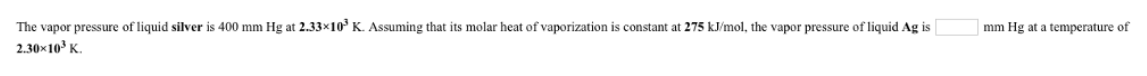# Problem: The vapor pressure of liquid silver is 400 mm Hg at 2.33 x 103 K. Assuming that its molar heat of vaporization is constant at 275 kJ/mol, the vapor pressure of liquid Ag is _______ mm Hg at a temperature of 2.30 x 103 K.

###### FREE Expert Solution
86% (313 ratings)###### Problem Details

The vapor pressure of liquid silver is 400 mm Hg at 2.33 x 103 K. Assuming that its molar heat of vaporization is constant at 275 kJ/mol, the vapor pressure of liquid Ag is _______ mm Hg at a temperature of 2.30 x 103 K.Frequently Asked Questions

What scientific concept do you need to know in order to solve this problem?

Our tutors have indicated that to solve this problem you will need to apply the Clausius-Clapeyron Equation concept. You can view video lessons to learn Clausius-Clapeyron Equation. Or if you need more Clausius-Clapeyron Equation practice, you can also practice Clausius-Clapeyron Equation practice problems.

What professor is this problem relevant for?

Based on our data, we think this problem is relevant for Professor Gu's class at SDSU.